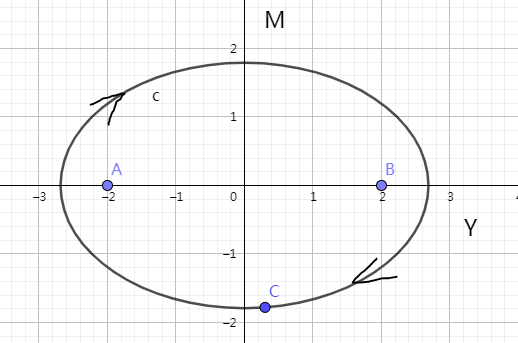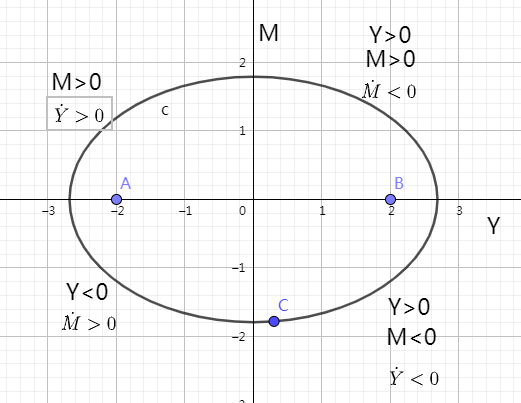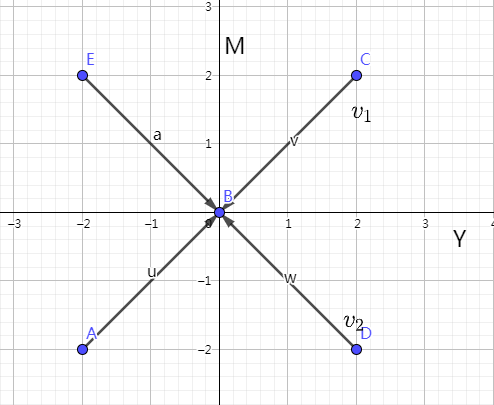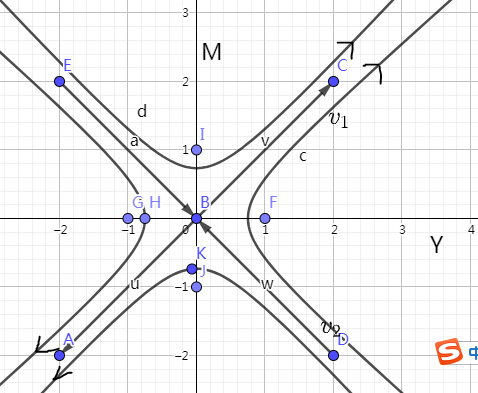Y

(

t

)

Y(t)

Y

>

0

Y>0

Y

<

0

Y<0

M

(

t

)

M(t)

M

>

0

M>0

M

<

0

M<0

# case 1

Y

˙

=

a

M

\dot{Y} = aM

M

˙

=

b

Y

\dot{M} = -bY

a

,

b

>

0

a,b>0

，可以得出平衡点为

Y

f

=

0

,

M

f

=

0

Y_f=0,M_f=0

，在进行分析之前，我们先来描述一下这个系统。

step1：系统描述
（1）根据这个函数表达式，可以看出与非是个耿直boy，你对我好，我也对你好；你讨厌我，我也不理你。也就是投桃报李+以牙还牙的性格
（2）梦寒则是一个多情的girl，你越热情她越远离；你越冷淡她越着迷，是欲迎还拒+若即若离的性格

step2：计算

[

Y

˙

M

˙

]

=

[

0

a

b

0

]

[

Y

M

]

\left[ \right] =\left[ \right] \left[ \right]

A

A

A

A

λ

I

A

=

0

|\lambda I-A|=0

，可以求出

λ

=

±

a

b

i

\lambda=\pm\sqrt{ab}i

。我们知道这是一个center，在直角坐标系中可以表示成一个圆。step3：分析

Y

>

0

Y>0

M

<

0

M<0

，同时

Y

˙

<

0

\dot{Y}<0

，说明与非的热情在减少当中，当移动到临界点的时候，与非开始讨厌梦寒了，此时

Y

<

0

Y<0

M

˙

>

0

\dot{M}>0

，也就是梦寒对与非的状态发生了改变，然后到了第二象限，梦寒开始喜欢与非了(

M

>

0

M>0

)，而随着梦寒的亲近，与非的热情又被点燃了起来，直到某一刻（进入第一象限），与非又开始喜欢梦寒了，但此时的梦寒呢，又受不了与非的热情，开始变得冷淡了起来，他们就这样无限循环，爱恨交织下去。step4:讨论

# case 2

Y

˙

=

a

Y

+

b

M

\dot{Y} = -aY+bM

M

˙

=

b

Y

a

M

\dot{M} = bY-aM

a

,

b

>

0

a,b>0

，可以看出平衡点也都是0。

step1：系统描述

Y

,

M

Y,M

b

>

0

b>0

），同时他们都很小心，都有所保留（对自己都是负值，

a

<

0

-a<0

）。

step2：计算

A

A

A

=

[

a

b

b

a

]

A = \left[ \right]

λ

1

=

a

+

b

,

λ

2

=

a

b

\lambda_1 = -a+b, \lambda_2 = -a-b

，特征向量为

v

1

=

[

1

,

1

]

,

v

2

=

[

1

,

1

]

v_1 = [1, \ 1], \ v_2 = [1, \ -1]

step3:分析

• case2.1

a

>

b

|a|>|b|

，此时

λ

1

<

0

,

λ

2

<

0

\lambda_1<0,\ \lambda_2<0这是一个sink点，随着时间t趋近于无穷，

Y

Y

M

M

终将会变成路人。up主说“十年之后，我们是朋友，还可以问候，却再也找不到拥抱的理由”。
• case2.2

a

<

b

|a|<|b|

，此时

λ

1

>

0

,

λ

2

<

0

\lambda_1>0, \ \lambda_2<0v

2

v_2

向量上方的一点相遇，此时

Y

>

0

,

M

<

0

Y>0,M<0

，与非深爱着梦寒，梦寒却有点讨厌与非，在之后的交往中，梦寒逐渐升温（M变大），与非虽然动摇过（Y先减小后增大），但却一直爱着她，这样，终于突破临界点，两个人开始过上了幸福的生活。
如果说说在第四象限

v

2

v_2

向量下方的一点相遇，此时

Y

>

0

,

M

<

0

Y>0,M<0

，与非深爱着梦寒，梦寒非常讨厌与非，在之后的交往中，梦寒逐渐升温，但与非在交往中坚持不了崩溃了，最后两人形同陌路。
step4：讨论
我们来讨论一下这两种结果，当

a

>

b

|a|>|b|

的时候，我们实际上在说一个人的自我意识要大于对对方的感受，最后两个人要变成路人，

a

<

b

|a|<|b|

的时候，是说对对方的感受要大于自我意识，这时候会有两种结果，要么共浴爱河，要么因爱生恨。这就验证了很多人宁愿做朋友，也不愿意去打破平衡，也说明了你不认真肯定是赢不了的，但认真的话你可能就输了。